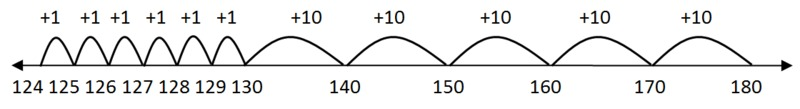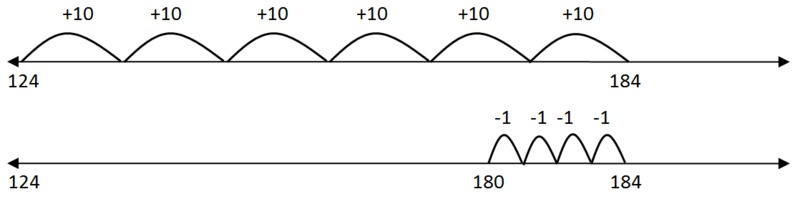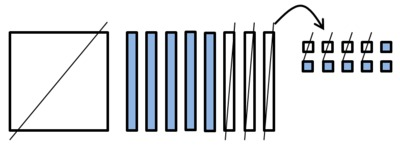# 2.NBT How Many Days Until Summer Vacation?

#### Materials

• Paper
• Pencil
• Hundreds board
• Base-ten blocks

#### Actions

Pose this problem to the children:

We are in school 180 days. Today is the 124th day of school. How many more days until we are out of school for summer vacation? Explain how you know.

### Commentary

The purpose of the task is to allow children an opportunity to subtract a three-digit number including a zero that requires regrouping. The solutions show how students can solve this problem before they have learned the traditional algorithm. Children need to be familiar with the 100s board, base ten blocks, counting on, and counting backwards. The solutions given make sense to children and are often easier for them to explain and justify than using the traditional algorithm.

The complexity of this task can be modified depending on the day such a problem is given. For example, on the 110th day of school, the subtraction is very simple: once students can count by tens, they can easily solve it. This task could also be used to give students extra practice outside of math instructional time; for example, if teachers go over the calendar at some point during the day, such a problem could be given then as a way to get them doing math, even though they're not in math time.

### Solutions

Solution: 1

Count by ones to the next decade: 125, 126, 127, 128, 129, 130. So we have added 6.

Then count by tens: 140, 150, 160, 170, 180, which makes 5 tens or 50.

Since 50 + 6 = 56, there are 56 days left before we get out of school for summer vacation.

Students can use the 100s board or draw an "empty number line" to help them count.Solution: 2

Count by tens from 124: 134, 144, 154, 164, 174, 184. That is 6 tens or 60.

But 184 is too big so count back to 180, which is 4.

Subtract 4 from 60 to get 56. We have 56 days of school left.Solution: 3

Using base-ten blocks:

Start with 1 hundred, 8 tens, 0 ones. We can’t take away 4 ones from 0 ones so we have to break a ten into 10 ones. I now have 7 tens 10 ones. Now we can subtract using the take-away model.

10 - 4 = 6.

70 - 20 = 50.

100 - 100 = 0.

So there are 56 days of school before we get out for summer vacation. Here is a picturing showing the 100s, 10s and 1s.